# The SEQTEST Procedure

### Available Sample Space Orderings in a Sequential Test

Subsections:

At the end of a trial, the hypothesis is either rejected or accepted. Denote the stage number and the statistic at the end of a trial by a pair of statistics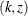, where k is the stage number and z is the standardized Z statistic. Then an ordering on the sample spaceis needed to derive the p-value, median, and confidence limits associated with the observed statistics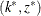.

The SEQTEST procedure provides the stagewise, LR, and MLE orderings. See Jennison and Turnbull (2000, pp. 179–187) for a detailed description and comparison of these orderings.

#### Stagewise Ordering

If the continuation regions of a design are intervals, the stagewise ordering (Fairbanks and Madsen 1982; Tsiatis, Rosner, and Mehta 1984; Jennison and Turnbull 2000, pp. 179–180) uses counter-clockwise ordering around the continuation region to compute the p-value, unbiased median estimate, and confidence limits. This ordering depends on the stopping region, stopping stage, and standardized statistic at the stopping stage. But it does not depend on information levels beyond the observed stage. For a one-sided design with an upper alternative,if one of the following criteria holds:

•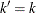and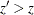•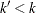and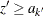, the upperboundary at stage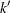•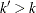and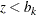, the upperboundary at stage k

Similar criteria can be derived for a one-sided design with a lower alternative.

For a two-sided design with early stopping to reject the null hypothesis,if one of the following criteria holds:

•and•and, the upperboundary at stage•and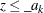, the lowerboundary at stage k

Note that the stagewise ordering is not applicable for two-sided designs with early stopping to acceptor to either accept or reject, which might have two disjoint continuous intervals at each interim stage.

For a two-sided design with early stopping either to reject or to accept the null hypothesis,if one of the following criteria holds:

•and•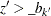andThat is, each value in the continuation region is less extreme than each value in the upper rejection region and more extreme than each value in the lower rejection region. Then, combining with the ordering defined for a two-sided design with early stopping to reject the null hypothesis, the p-value, median, and confidence limits can be derived for the observed statistics in the lower or upper rejection region.

Thus, if the stagewise ordering is specified in the SEQTEST procedure for a two-sided design with early stopping to either reject or accept the null hypothesis, the stagewise ordering is used to derive these statistics only if the observed statistics is in the lower or upper rejection region. Otherwise, the LR ordering is used.

#### LR Ordering

The LR ordering (Chang, 1989) depends on the observed standardized Z statistic z, information levels, and a specified hypothetical reference. For the LR ordering under a given hypothesis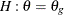,if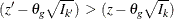Under the null hypothesis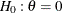, it reduces to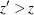and can be used to derive statistics under, such as p-values.

The LR ordering is applicable to all designs if all information levels are available. But depending on the boundary shape, some observed statisticsin the rejection region might be less extreme than the statistics in the acceptance region. That is, the p-value for observed statistics in the rejection region might be greater than the significance level.

#### MLE Ordering

The MLE ordering (Emerson and Fleming, 1990) depends only on the observed maximum likelihood estimate.if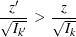The MLE ordering is applicable to all designs if all information levels are available.